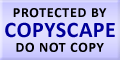Submit Assignment From Here
Name
:
Email
:
Phone
:
Country
:
Willing to pay US\$
:
Subject
:
Topic
:
Levels
:
:Hours
:

# Regression Homework Help

Regression is a statistical analysis which measures the relationship between two or more variables. There are many techniques which analyzes the relationship between a dependent variable and an independent variable. Regression analysis helps the user understand the relation between the dependent and independent variables. When an independent variable is changed how the value of dependent variable changes by the impact of change in that variable which is independent on the other side all other variables remain constant. So we understand that regression analysis is the method by which user is able to understand the expected change in the average value of the dependent variable when independent variables are constant.
Regression analysis is basically used in forecasting and making predictions. The regression analysis is performed on the basis of data generation. As the original data generation process is not available there are assumptions taken to generate data. Regression model has the following variable:
Z is the independent variable, α is the unknown parameter, and X is the dependent variable. Thus we can form a model equation in the following manner:
X ≈f ( Z,α)
Where the no. of unknown parameters is lesser then the no. of measurements and the measurement errors are normally distributed then the excess of information is used to make predictions. This is known as degree of freedom of regression.Make Payment OnlineUS